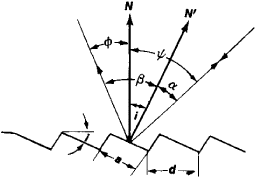# Echelette

The following article is from The Great Soviet Encyclopedia (1979). It might be outdated or ideologically biased.

## Echelette

(also echelette grating), a diffraction grating of the reflection type that can concentrate diffracted radiation in a spectrum of one order while attenuating spectra of other orders, including the brightest spectrum of zeroth order (seeORDER OF INTERFERENCE). Both the spectral distribution of the radiation and the high concentration of energy in a narrow spectral region are varied by the introduction of an additional path difference within each individual groove, which, as a rule, has a triangular profile.

Reflection gratings of the echelette type are usually engraved on a metal surface—for example, copper, brass, or aluminum—by means of special ruling engines. Such gratings are used to observe spectra of fifth to tenth order in the infrared region. Eche-lettes may also be produced for the visible and ultraviolet spectral regions.

An echelette is a system of identical mirror surfaces (see Figure 1) of width a whose planes are parallel to one another and subtend an angle i with the plane of the grating. When a parallel beam of rays is incident on an echelette, the beam is diffracted by each mirror surface, as if by a narrow slit; beams that are diffracted by all the mirror surfaces interfere. Radiant energy is concentrated in a given direction if the following conditions are met: (1) the direction from an individual groove to the zeroth-order maximum is the same as the direction from the entire grating to the central maximum and (2) the direction to the zeroth-order spectrum of the entire grating is the same as the direction of the minimum upon diffraction by an individual groove.

Condition (1) means that the direction φ in the equation for the maximums in the case of a reflection grating, d (sin ψ + sin φ) = , should be the same as the angle β = – α. Bearing in mind the sign convention and taking into account relations of the type φ = i – α and ψ = i + α, we obtain the expression 2 cos (ψ – i) sin i = nλ/d. This expression makes it possible to calculate the angle of inclination i of a mirror surface from a given angle of incidence ψ and a given wavelength λ The angle i is called the blaze angle and ranges from 5° to 20° in present-day echelettes.

Condition (2) means that, for the zeroth-order spectrum, that is, for ψ = – φ, the direction to the spectrum should be the sameFigure 1. Diagram of a portion of the surface of an echelette and the path of rays incident on and diffracted by the echelette: (a) width of the mirror surface of a groove, (d) grating constant, (N) normal to the surface of the echelette, (N′) normal to the mirror surface of the groove, (i) blaze angle, (ψ) angle of incidence of the rays on the echelette, (φ) angle of emergence, (α) angle of incidence of the rays on the mirror surface of the groove, (β) angle of emergence from the mirror surface

as the direction β in the equation for the minimums upon diffraction by an individual mirror surface: α(sin α + sin β) = kλ. When the relation – β = ψ + i is taken into account, the expression 2 sin i cos ψ = kλ/a is obtained. For a known blaze angle i, this expression makes it possible to calculate the width a of a mirror surface.

If conditions (1) and (2) are met, the maximum of the energy reflected from the grating is located in the direction φ = 2i – ψ, which is the same as the direction of specular reflection from the plane of a groove. Reflection gratings are used most often in autocollimators, for which ψ = φ = i From the equation for the maximums in this case, the wavelength to which the maximum concentration of energy corresponds is easily obtained: nλmax = 2d sin i. The wavelength range near λmax is the range of high energy concentration in a given order of interference.

In a spectrum of a single order, present-day echelettes concentrate up to 70–80 percent of the energy of the incident radiation. The use of echelettes makes it possible to construct spectroscopic instruments that are not inferior in light power to the best instruments based on dispersing prisms.

In the USSR, echelettes are manufactured in which the number of grooves, or lines, ranges from 600 per mm for the visible region to 0.3 per mm for the far-infrared region (wavelengths of ~500 micrometers). The size of the echelettes ranges from 100 × 100 mm for gratings with 100–300 lines per mm to 300 × 300 mm for gratings with 12 lines per mm or less.

### REFERENCES

Peisakhson, I. V. Optika spectral’nykh priborov. Leningrad, 1975.
Nagibina, I. M. Interferentsiia i difraktsiia sveta. Leningrad, 1974.
Kaliteevskii, N. I. Volnovaia optika. Moscow, 1971.

L. N. KAPORSKII